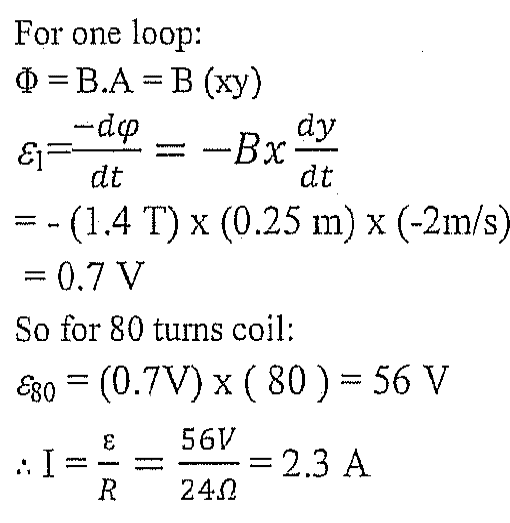# Magnetic Flux

## Homework Statement

Uploaded the question below , Part B

## Homework Equations

Φ=NBA
EMF= (delta)Φ/(delta)t
V=IR

## The Attempt at a Solution

so i found the area (0.25x0.3) and then divided by two (=0.0375m^2) , then found Φ=80x1.4x0.0375=4.2wb
However , in the marking scheme they multiplayed by the speed instead of area !!#### Attachments

BvU
Homework Helper
So, you calculated ##\Phi##. But for the emf you need ##d\Phi\over dt##. Is the step from one to the other unclear to you ?

So, you calculated ##\Phi##. But for the emf you need ##d\Phi\over dt##. Is the step from one to the other unclear to you ?
i know i have to use dΦ/dt , thats why i calculated Φ .. couldnt get how they found 0.7v

BvU
Homework Helper
Area with magnetic field within the loop is xy . x is constant, 0.25 m. At t=0 y = 0.15 m . When the coil is moving, y decreases by 2 m/s

Area with magnetic field within the loop is xy . x is constant, 0.25 m. At t=0 y = 0.15 m . When the coil is moving, y decreases by 2 m/s
so its like we make change of "y" over time equals to the speed ? since speed = distance covered / time taken

BvU